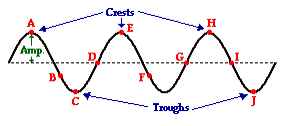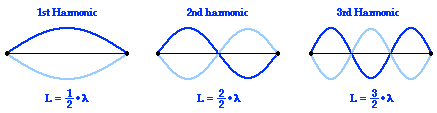# Waves, Sound and Light: Wave Basics

## Wave Basics: Problem Set Overview

This set of 29 problems targets your ability to determine wave quantities such as frequency, period, wavelength, amplitude and speed from verbal descriptions and diagrams of physical situations pertaining to waves. Problems range in difficulty from the very easy and straight-forward to the very difficult and complex. The more difficult problems are color-coded as blue problems.

## Frequency and Period

A wave is an example of periodic motion. The particles of the medium oscillate back and forth about a fixed position. The time which it takes for such a particle to complete one full cycle is known as the period. Period units are simply seconds (or minutes or hours or some time unit). The frequency of a wave describes the number of complete cycles which are completed during a given period of time. As such, frequency is a rate quantity which describes the rate of oscillations or vibrations or cycles or waves on a per second basis. A common unit of frequency is the Hertz, abbreviated as Hz. A Hertz indicates the number of complete cyles per second.

There is a mathematical relationship between the frequency (f) of the wave and the period (T) of the wave as denoted by the following relationship:

f = 1 / T

Knowing frequency allows one to automatically calculate the period, and vice versa.

## Wave Speed

Waves propagate through a medium. The familiar pattern of the sine wave consists of a crest and a trough (or in the case of longitudinal waves, a compression and a rarefaction). The crest can be seen moving along the medium from one location to another location. The speed (or velocity) of a wave is defined as the distance a crest on the wave travels per unit of time. Like the speed of a runner or a car, wave speed (v) is simply the ratio of the distance (d) traveled per time of travel (t) as shown by the following equation.

v = d / t

## The Anatomy of a Wave

Imagine a wave moving through a medium such as a rope or snakey. And then imagine that a snapshot could be taken at an instant in time, thus freezing the motion of the wave and providing a picture of the shape of the medium. The result would be a diagram similar to that below (of course, without all the labels).The labels have been added to indicate a few strategic points. The dashed line which stretches horizontally across the middle of the pattern is known as the rest position. The points labeled crests are at the points of maximum displacement above the rest position; the troughs are at the points of maximum displacement below the rest position. The vertical distance from a crest to the rest position is known as the amplitude. And the horizontal distance stretching between any two adjacent crests (or between adjacent troughs) is known as the wavelength. A more detailed and exhaustive discussion of the anatomy of a wave can be found at The Physics Classroom Tutorial.

## The Wave Equation

There is a mathematical relationship between the speed or velocity (v) of a wave and the frequency (f) and wavelength (λ) of the wave. That relationship is expressed by the wave equation

v = f • λ

Knowledge of two of the three quantities allows one to calculate the third quantity.

## Standing Wave Pattern Analysis

When a wave is introduced into a medium such as a rope or a snakey, it travels the length of the medium and then reflects back upon reaching the end of the medium. At certain frequencies, the reflected portion of the wave meets up with the original wave to create a pattern known as a standing wave pattern. In a standing wave pattern there are points along the medium which appear as if they are always standing still. These points are known as nodes and are easily remembered as the points of no displacement. There is always an antinode positioned between two adjacent nodes. Antinodes are points of maximum positive and negative displacement. In such standing wave patterns, there is a unique half-number relationship between the length of the medium and the wavelength of the waves which have established the pattern which is present in the medium. These relationships are shown below for the standing wave patterns having one node (first harmonic), two nodes (second harmonic) and three nodes (third harmonic).Other patterns for four nodes and more could be drawn by simply extending the principles which have been used for the first three patterns. From the pattern and knowledge of the length of the medium, the wavelength can be determined using the above equations. Similarly, the length of the medium can be determined from the wavelength. A more detailed and exhaustive discussion of the mathematics of standing wave patterns can be found at The Physics Classroom Tutorial.

## Wave Interference

The meeting up of two waves while traveling in opposite directions along the same medium is known as interference. When two waves interfere, the medium takes on a new resultant shape which reflects the simultaneous influence of both waves upon the particles of the medium. The amount of displacement of the individual particles can be determined using the principle of superposition. According to this principle, the displacement of an individual particle at a moment in time is simply the algebraic sum of the displacements that the particle would have as a result of the individual waves. So if wave A has a crest which meets up with a trough of wave B at a given location and a specified moment in time, then the displacement of the medium at that location is simply the sum of wave A's amplitude and wave B's amplitude. In this case, Wave B would have a negative amplitude since it is a trough; and so a positive amplitude and a negative amplitude would be added together to determine the displacement of the medium at that location and moment in time. A more detailed and exhaustive discussion of wave interference and the principle of superposition can be found at The Physics Classroom Tutorial.

## Boundary Behavior

Mechanical waves travel through a medium (water, string, rope, snakey). The medium does not extend through space forever; eventually a medium ends. For instance, a rope may be terminated at wall to which it is tied, thus forming a boundary with a different medium (the wall). Or the end of a snakey may be tied to the end of another snakey, thus forming a boundary between two snakeys. As a wave reaches such a boundary, a portion of its energy is transmitted into the new medium and a portion of its energy is reflected off the boundary back into the original medium. This is known as boundary behavior. As a result of the reflection and transmission, changes in amplitude, wavelength and speed can often be observed. Most commonly, one observes that a transmitted wave (or pulse) has a different wavelength and speed than the incident wave (or pulse). While wavelength and speed may change as a wave is transmitted across a boundary, the frequency remains the same. Knowing this allows one to conduct a boundary analysis for the transmission of a wave across the boundary. A more detailed and exhaustive discussion of boundary behavior can be found at The Physics Classroom Tutorial.

## Using a Concepts Based Approach

Physics problems are more than mere exercises in the mathematical manipulations of algebra formulas. Make no mistake about it, the solution to many physics problems requires an ability to mathematically manipulate an equation in order to solve for an unknown variable. However, many physics problems require more than mathematics. In fact, in many instances a student exercising good mathematical skills misses a problem because of a conceptual misunderstanding. In many of the problems in this set, you will need to use your understanding of wavelength, frequency, period and wave speed to appropriately interpret the verbal information in the problem statement. For instance, you will have to read a phrase such as "vibrating it up and down with 32 vibrations in 10 seconds" and use conceptual understandings to appropriately extract the numerical information from the problem and associate it with variables of the equations. Furthermore, there are many instances in which extraneous numerical values are stated in the problem description; such values do not need to be used in the solution. This extraneous information will only be a distraction to students who treat physics problems as mere mathematical exercises with little connection to physics concepts.

Physics is about conceptual ideas and relationships; and problem sets test your conceptual understanding of these relationships. If you treat this problem set as a mere exercise in the algebraic manipulation of physics equations, then you are likely to become frustrated quickly. As you proceed through this problem set, be concepts-minded. Never strip physics of its conceptual meaning.

## Habits of an Effective Problem-Solver

An effective problem solver by habit approaches a physics problem in a manner that reflects a collection of disciplined habits. While not every effective problem solver employs the same approach, they all have habits which they share in common. These habits are described briefly here. An effective problem-solver...

• ...reads the problem carefully and develops a mental picture of the physical situation. If needed, they sketch a simple diagram of the physical situation to help visualize it.
• ...identifies the known and unknown quantities in an organized manner, often times recording them on the diagram iteself. They equate given values to the symbols used to represent the corresponding quantity (e.g., v = 12.8 m/s, λ = 4.52 m, f = ???).
• ...plots a strategy for solving for the unknown quantity; the strategy will typically center around the use of physics equations be heavily dependent upon an understanding of physics principles.
• ...identifies the appropriate formula(s) to use, often times writing them down. Where needed, they perform the needed conversion of quantities into the proper unit.
• ...performs substitutions and algebraic manipulations in order to solve for the unknown quantity.

The following pages from The Physics Classroom tutorial may serve to be useful in assisting you in the understanding of the concepts and mathematics associated with these problems.

# Problem Sets and Audio Guided Solutions

## Wave Basics Audio Guided Solutions

View the audio guided solution for problem:
1 | 2 | 3 | 4 | 5 | 6 | 7 | 8 | 9 | 10 | 11 | 12 | 13 | 14 | 15 | 16 | 17 | 18 | 19 | 20 | 21 | 22 | 23 | 24 | 25 | 26 | 27 | 28 | 29